## 第二次随笔作业

##### 7-7 计算火车运行时间 （15 分）

#include<stdio.h>
int main()
{
int a,b,c,d,e,f,x,y;
scanf("%d %d",&x,&y);
a=x/100;b=x%100;
c=y/100;d=y%100;
if(b<=d){
e=c-a;f=d-b;
}
else{
e=c-1-a;f=d+60-b;
}
printf("%02d:%02d",e,f);
return 0;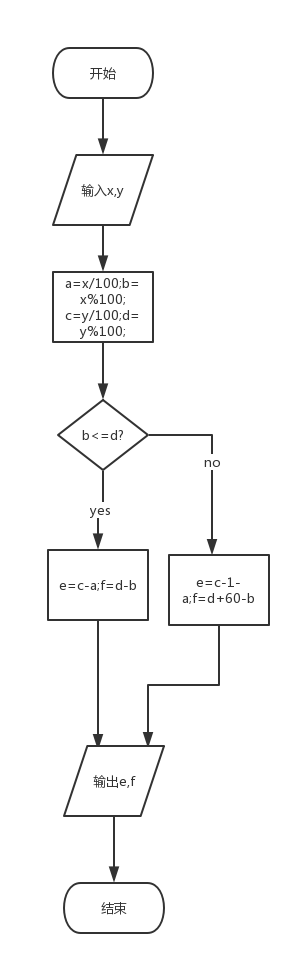}

这是我调试时输入第一个变量时的截图：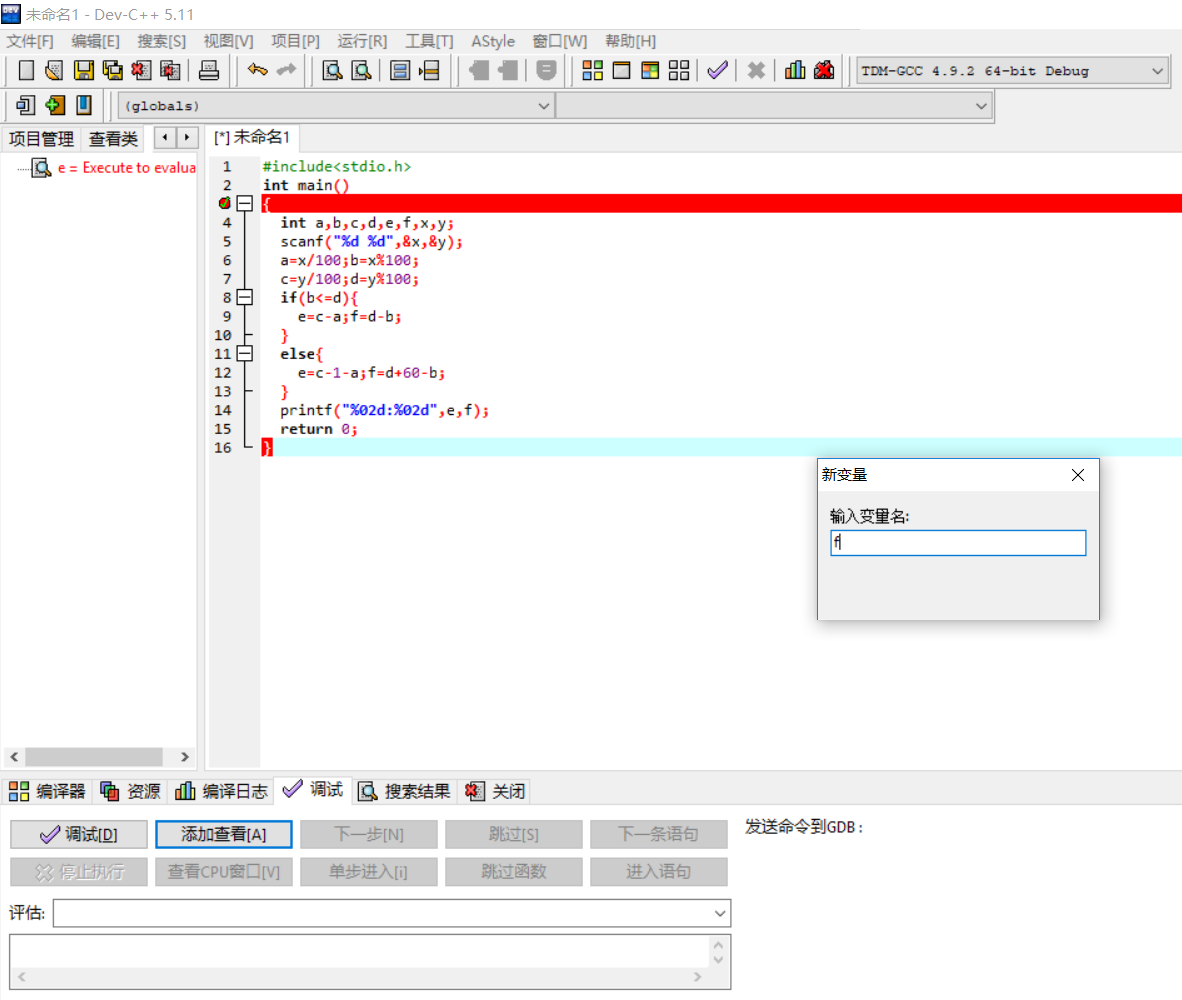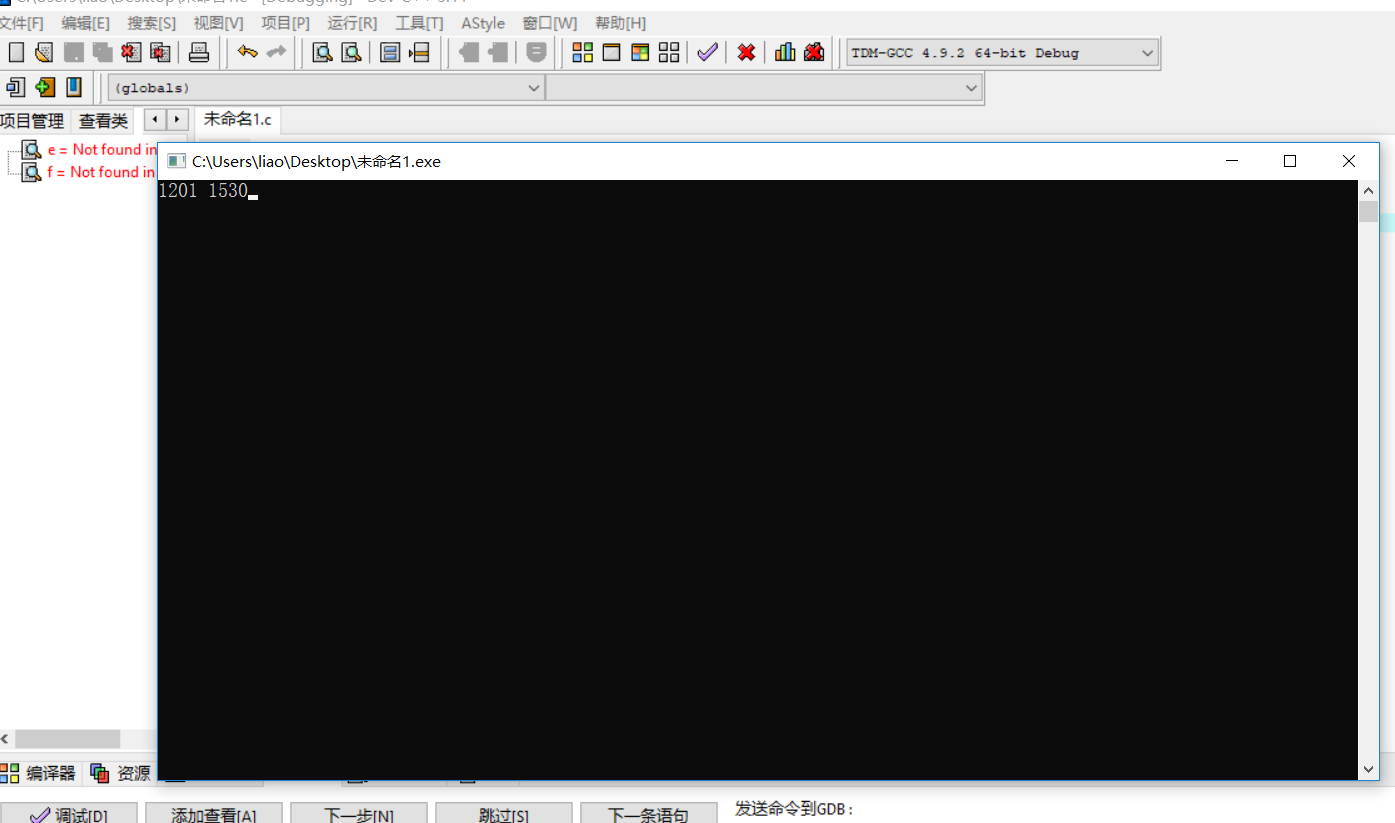a大则交换值，确保a是最小数，

##### 7-4 比较大小 （10 分）

#include<stdio.h>
int main(void)
{
int a,b,c,d;
scanf("%d %d %d",&a,&b,&c);
if(a>=b){
d=a;a=b;b=d;
}
if(a>=c){
d=a;a=c;c=d;
}
if(b>=c){
d=b;b=c;c=d;
}
printf("%d->%d->%d",a,b,c);
return 0;
}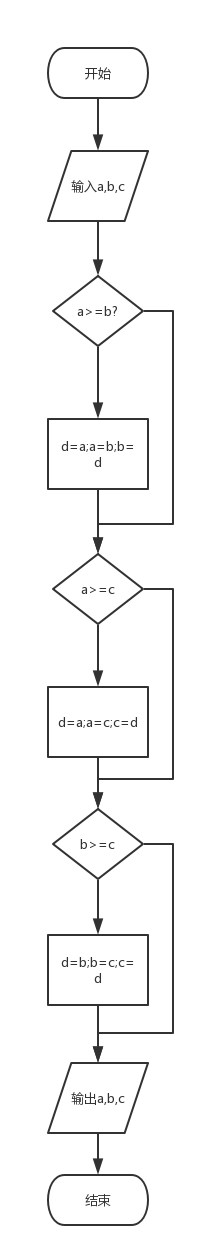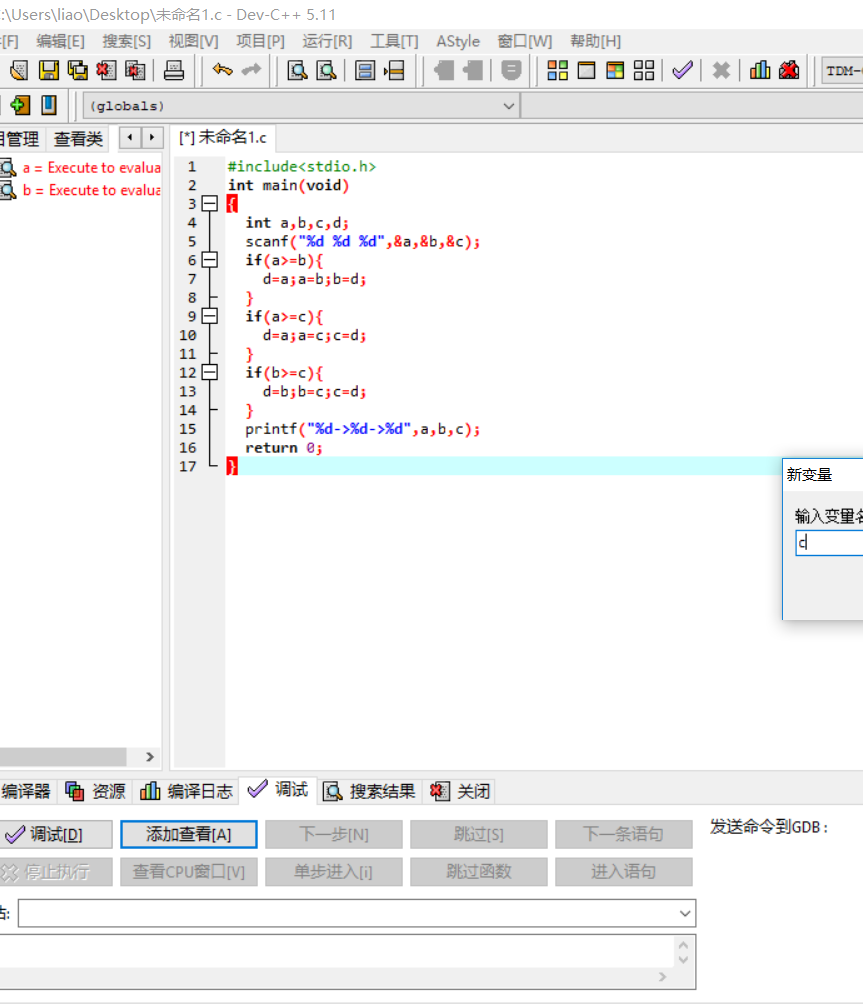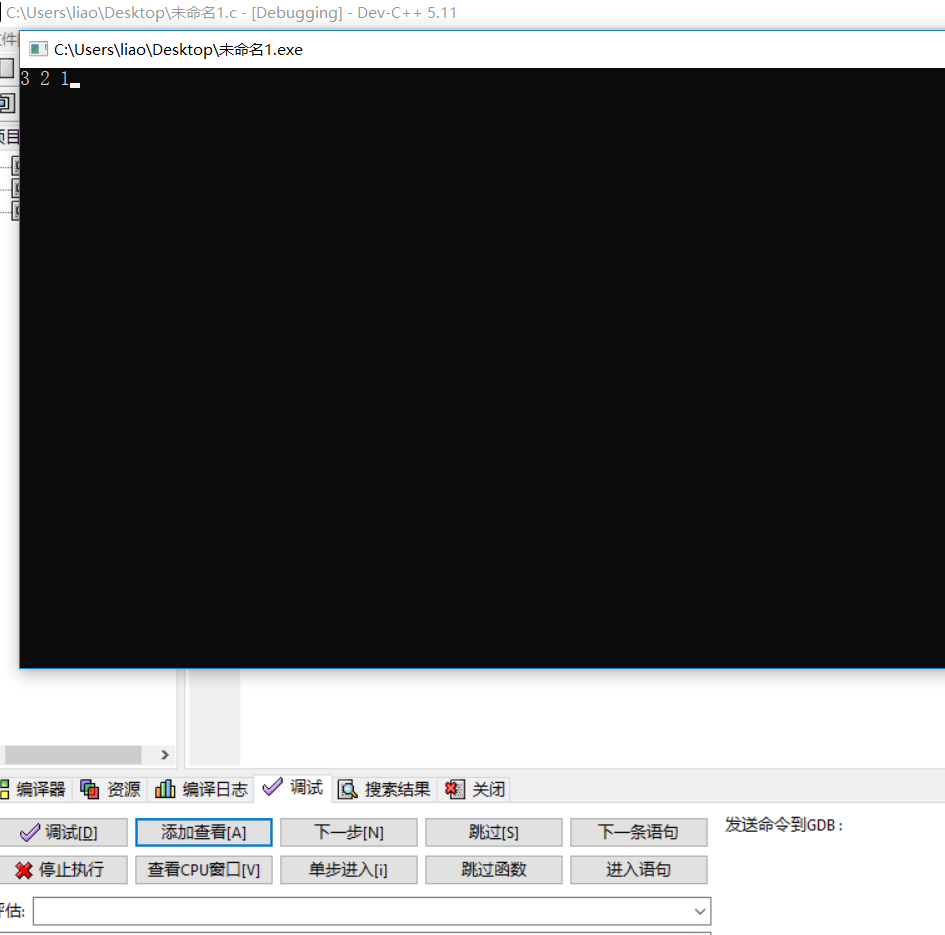要求三：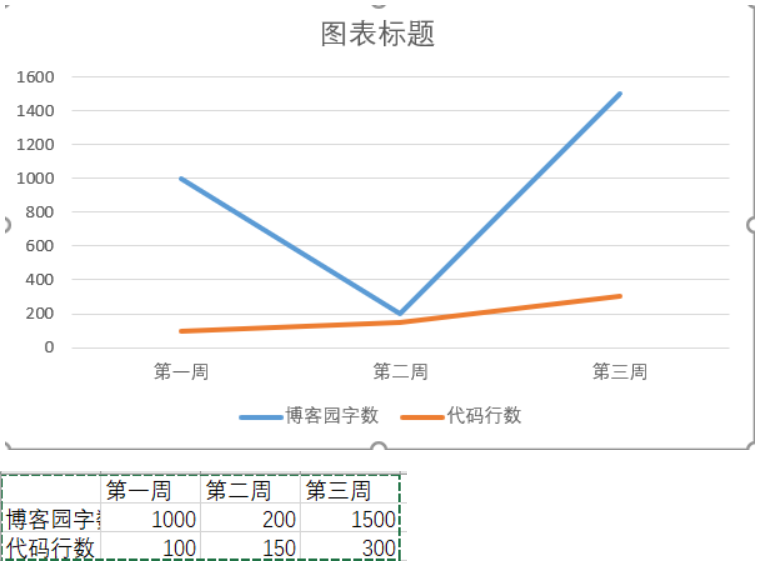posted on 2018-11-06 13:17  张博张博  阅读(164)  评论(4编辑  收藏  举报